Skip to main content

# A fractional order nonlinear dynamical model of interpersonal relationships

## Abstract

In this paper, a fractional order nonlinear dynamical model of interpersonal relationships has been introduced. The stability of equilibrium points is studied. Numerical simulations are also presented to verify the obtained results.

## 1 Introduction

In recent decades the study of interpersonal relationships has begun to be popular. Interpersonal relationships appear in many contexts such as family, kinship, acquaintance, work, and clubs . Mathematical modeling in interpersonal relationships is very important for capturing the dynamics of people. But there are few models in this area, and models have been restricted to integer order differential equations. Since experiments in this area are difficult to design and may be constrained by ethical considerations, mathematical models can play a vital role in studying the dynamics of relationships and their behavioral features . In this paper, we consider a system of nonlinear fractional differential equations. This fractional system of equations is obtained by replacing a derivative term by a fractional derivative of order $α>0$. The integer order model reported in  is given as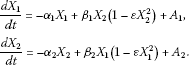A fractional order system instead of its integer order counterpart has been considered because fractional order differential equations are generalizations of integer order differential equations and fractional order models possess memory. Also, the fact that interpersonal relationships are influenced by memory makes fractional modeling appropriate for this kind of dynamical systems .

In this paper, firstly a fractional order nonlinear dynamical model of interpersonal relationships has been introduced. A detailed analysis for the asymptotic stability of equilibrium points has been given. Finally, numerical simulations are presented to verify the obtained results.

## 2 Model

First of all, we recall the definitions of fractional order integrals and derivatives .

Definition 1 The Riemann-Liouville type fractional integral of order $α>0$ for a function $f:(0,∞)→R$ is defined by

$I α f(t)= 1 Γ ( α ) ∫ 0 t ( t − τ ) α − 1 f(τ)dτ.$

Here and elsewhere $Γ(⋅)$ denotes the gamma function.

Definition 2 The Riemann-Liouville type fractional derivative of order $α>0$ for a function $f:(0,∞)→R$ is defined by

$D α f(t)= d n d t n 1 Γ ( n − α ) ∫ 0 t ( t − τ ) n − α − 1 f(τ)dτ,$

where $n=⌈α⌉+1$ and $⌈α⌉$ is the integer part of α.

Definition 3 The Caputo-type fractional derivative of order $α>0$ for a function $f:(0,∞)→R$ is defined by

$D α f(t)= 1 Γ ( n − α ) ∫ 0 t ( t − τ ) n − α − 1 f n (τ)dτ,$

where $n=⌈α⌉+1$ and $⌈α⌉$ is the integer part of α.

Generally, in mathematical modeling we use Caputo’s definition. The main advantage of Caputo’s definition is that the initial conditions for fractional differential equations with Caputo derivatives take on the same form as for integer-order differential equations .

The model that we study in this paper is a fractional order nonlinear dynamical model of interpersonal relationships. The model is a nonlinear dynamical system with two state variables. The variables $X 1$ and $X 2$ are the measures of love of individuals 1 and 2 for their respective partners, where positive and negative measures represent feelings. The following model is considered:

$D α X 1 ( t ) = − α 1 X 1 + β 1 X 2 ( 1 − ε X 2 2 ) + A 1 , D α X 2 ( t ) = − α 2 X 2 + β 2 X 1 ( 1 − ε X 1 2 ) + A 2$
(1)

with initial conditions

$X 1 (0)= X 01 , X 2 (0)= X 02 ,$
(2)

where $0<α≤1$, $α i >0$, $α i$, $β i$, and $A i$ ($i=1,2$) are real constants. These parameters are oblivion, reaction, and attraction constants. In the equations above, we assume that feelings decay exponentially fast in the absence of partners. The parameters specify the romantic style of individuals 1 and 2. For instance, $α i$ describes the extent to which individual i is encouraged by his/her own feeling. In other words, $α i$ indicates the degree to which an individual has internalized a sense of his/her self-worth. In addition, it can be used as the level of anxiety and dependency on other person’s approval in romantic relationships. The parameter $β i$ represents the extent to which individual i is encouraged by his/her partner, and/or expects his/her partner to be supportive. It measures the tendency to seek or avoid closeness in a romantic relationship. Therefore, the term $− α i X i$ says that the love measure of i, in the absence of the partner, decays exponentially and $1 α i$ is the time required for love to decay.

## 3 Equilibrium points and their asymptotic stability

Let $α∈(0,1]$ and consider the system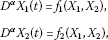with the initial values $X 1 (0)= X 01$ and $X 2 (0)= X 02$. Here $f 1 ( X 1 , X 2 )=− α 1 X 1 + β 1 X 2 (1−ε X 2 2 )+ A 1$ and $f 2 ( X 1 , X 2 )=− α 2 X 2 + β 2 X 1 (1−ε X 1 2 )+ A 2$.

To evaluate the equilibrium points, let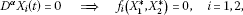from which we can get the equilibrium points $K 0 =(0,0)$ for $A 1 = A 2 =0$ and $K 1 =( X 1 ∗ , X 2 ∗ )$.

The Jacobian matrix $J( X 1 ∗ , X 2 ∗ )$ for the system given in (1) is

$J ( X 1 ∗ , X 2 ∗ ) = ∂ ( f 1 , f 2 ) ∂ ( X 1 ∗ , X 2 ∗ ) =[ ∂ f 1 ∂ X 1 ∗ ∂ f 1 ∂ X 2 ∗ ∂ f 2 ∂ X 1 ∗ ∂ f 2 ∂ X 2 ∗ ]=[ − α 1 β 1 ( 1 − 3 ε X 2 ∗ 2 ) β 2 ( 1 − 3 ε X 1 ∗ 2 ) − α 2 ].$

Mutual apathy equilibrium for the system given in (1) is $K 0 =( X 1 ∗ , X 2 ∗ )=(0,0)$ and the Jacobian matrix at this point is as follows:

$J(0,0)=[ − α 1 β 1 β 2 − α 2 ].$

Theorem 1 If one of the conditions below holds, the mutual apathy equilibrium $K 0 =(0,0)$ of the system given in (1) and (2) is asymptotically stable.

1. (i)

$1< − 4 β 1 β 2 ( α 1 − α 2 ) 2$,

2. (ii)

$β 1 β 2 α 1 α 2 <1$.

The equilibrium point $K 0 =(0,0)$ is otherwise unstable.

Proof The mutual apathy equilibrium is asymptotically stable if all of the eigenvalues, $λ i$, $i=1,2$ of $J( K 0 )$, satisfy the condition [5, 6]

$|arg λ i |> α π 2 .$
(3)

These eigenvalues can be determined by solving the characteristic equation

$det ( J ( K 0 ) − λ I ) =0,$

which leads to the equation

$λ 2 +Bλ+C=0,$

where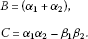The roots of the characteristic equation are

$λ 1 , 2 = − B 2 ± B 2 − 4 C 2 .$

The equilibrium point $K 0 =( X 1 ∗ , X 2 ∗ )=(0,0)$ is asymptotically stable if both the eigenvalues of the Jacobian matrix for the system given in (1) are negative ($|arg( λ 1 )|> α π 2$, $|arg( λ 2 )|> α π 2$). It is clear that $B=( α 1 + α 2 )>0$. If $B 2 −4C<0$, then all of the eigenvalues, $λ i$, $i=1,2$, are negative and satisfy the condition given by (i). If $B 2 > B 2 −4C$, then both of the eigenvalues, $λ i$, $i=1,2$, are negative and satisfy the conditions given by (ii). If one of the conditions above does not hold, model gives rise to unbounded feeling, which is obviously unrealistic. □

Theorem 2 We now discuss the asymptotic stability of the $K 1 =( X 1 ∗ , X 2 ∗ )$ equilibrium of the system given by (1). The Jacobian matrix $J( K 1 )$ evaluated at the $( X 1 ∗ , X 2 ∗ )$ equilibrium is given as

$J ( X 1 ∗ , X 2 ∗ ) =[ − α 1 β 1 ( 1 − 3 ε X 2 ∗ 2 ) β 2 ( 1 − 3 ε X 1 ∗ 2 ) − α 2 ].$

The characteristic equation of the linearized system is as follows:

$P(λ)= λ 2 +Dλ+K=0,$
(4)

where

$K = α 1 α 2 − β 1 β 2 ( 1 − 3 ε X 2 ∗ 2 ) ( 1 − 3 ε X 1 ∗ 2 ) , D = ( α 1 + α 2 ) .$
(4a)

The roots of the characteristic equation are

$λ 1 , 2 = − D 2 ± D 2 − 4 K 2 .$
(5)

The equilibrium point $K 1 =( X 1 ∗ , X 2 ∗ )$ of the system given in (1) and (2) is asymptotically stable if one of the following conditions holds for eigenvalues which are given as (5).

1. (i)

$1< − 4 β 1 β 2 ( α 1 − α 2 ) 2 (1−3ε X 2 ∗ 2 )(1−3ε X 1 ∗ 2 )$,

2. (ii)

$β 1 β 2 α 1 α 2 (1−3ε X 2 ∗ 2 )(1−3ε X 1 ∗ 2 )<1$.

The proof of Theorem 2 is similar to that of Theorem 1.

Theorem 3 Let $K= α 1 α 2 − β 1 β 2 (1−3ε X 2 ∗ 2 )(1−3ε X 1 ∗ 2 )$ be as given in (4a). If $K<0$, then the positive equilibrium point $K 1 =( X 1 ∗ , X 2 ∗ )$ of the system given in (1) and (2) is unstable.

Proof If $K<0$, from Descartes’ rule of signs, it is clear that the characteristic equation $P(λ)$ has at least one positive real root. So, the equilibrium point $K 1 =( X 1 ∗ , X 2 ∗ )$ of the system given in (1) and (2) is unstable. □

## 4 Numerical method

Consider the initial value problem (IVP) with Caputo-type FDE given by

$D α x ( t ) = f ( t , x ( t ) ) , x ( 0 ) = x 0 ,$
(6)

where $f∈C([0,T]×R,R)$, $0<α<1$. Since f is assumed to be a continuous function, every solution of IVP given by (6) is also a solution of the following Volterra fractional integral equation:

$x(t)= x 0 + 1 Γ ( α ) ∫ 0 t ( t − τ ) α − 1 f ( τ , x ( τ ) ) dτ,t∈[0,T].$
(7)

Moreover, every solution of (7) is a solution of IVP (6)  (Lakshmikantham and Vatsala 2007).

The following theorems are given for solving differential equations of fractional order in .

Theorem 4 Let $∥⋅∥$denote any convenient norm on $R n$. Assume that $f∈C[ R 1 , R n ]$, where $R 1 =[(t,X):0≤t≤a and ∥X− X 0 ∥≤b]$, $f= ( f 1 , f 2 , … , f n ) T$ and $X= ( x 1 , x 2 , … , x n ) T$, and let $∥f(t,X)∥≤M$ on $R 1$. Then there exists at least one solution for the system of FDE given by

$D α X(t)=f ( t , X ( t ) )$
(8)

with the initial condition

$X(0)= X 0$
(9)

on $0≤t≤β$, where $β=min(a, [ b M Γ ( α + 1 ) ] 1 α )$, $0<α<1$.

Theorem 5 Consider IVP given by (8)-(9) of order α ($0<α<1$). Let

$g ( ν , X ∗ ( ν ) ) =f ( t − ( t α − ν Γ ( α + 1 ) ) 1 / α , X ( t − ( t α − ν Γ ( α + 1 ) ) 1 / α ) )$

and assume that the conditions of Theorem 4 hold. Then a solution $X(t)$ of (6) can be given by

$X(t)= X ∗ ( t α / Γ ( α + 1 ) ) ,$

where $X ∗ (v)$ is a solution of the system of integer order differential equations

$d ( X ∗ ( ν ) ) d ν =g ( ν , X ∗ ( ν ) )$

with the initial condition

$X ∗ (0)= X 0 .$

## 5 Numerical solutions and simulations

Letting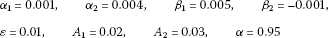we consider the system (1)-(2)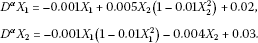(10)

Let the initial conditions be

$X 1 (0)=10, X 2 (0)=8.$
(11)

A positive equilibrium point for the problem (10)-(11) is calculated as

$X 1 ∗ =13.7604, X 2 ∗ =10.5737.$

For the numerical solution of (10)-(11), Theorem 5 has been used. The corresponding integer order system given in Theorem 5 is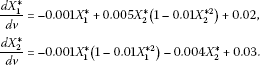If the solution of this integer order system is in $( X 1 ∗ (v), X 2 ∗ (v))$, then the solution of IVP (10)-(11) is $( X 1 ∗ ( t 0.95 /Γ(1.95)), X 2 ∗ ( t 0.95 /Γ(1.95)))$. We get the numerical solution of the integer order system by applying the fourth-order Runge-Kutta method. The approximate solutions $X 1 (t)$ and $X 2 (t)$ are displayed in Figure 1 for $α=0.95$. Figure 2 shows the asymptotic approximation of $( X 1 (t), X 2 (t))$ to the equilibrium point $(13.7604,10.5737)$.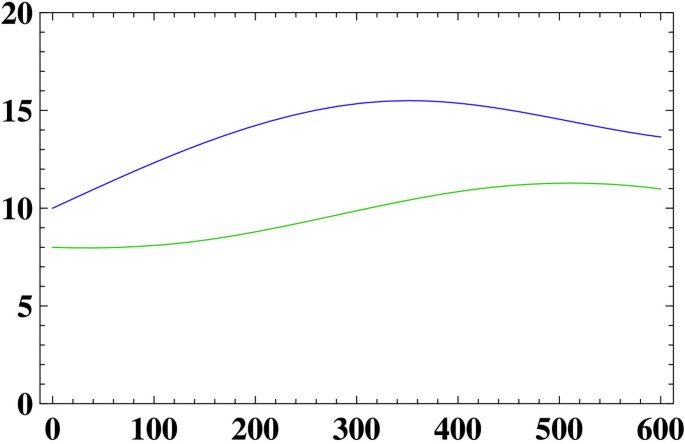Figure 1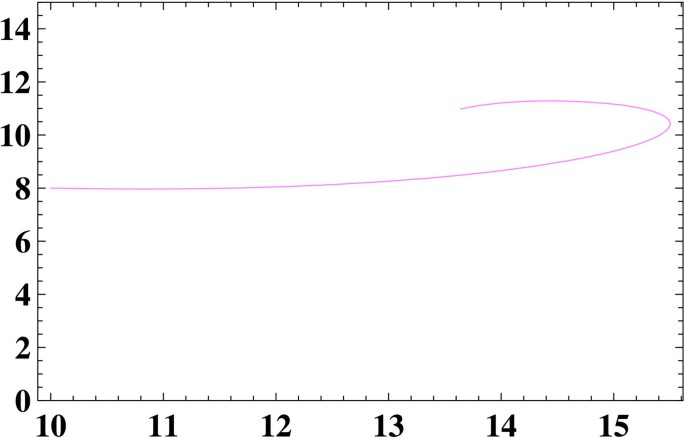Figure 2

## 6 Concluding remarks

We have formulated and analyzed a fractional order nonlinear dynamical model of interpersonal relationships. We have obtained a stability condition for equilibrium points. We have also given a numerical example and verified our results.

## References

1. 1.

Cherif A: Stochastic Nonlinear Dynamical Models of Interpersonal and Romantic Relationships: Strange Attractions. Arizona State University, Temple; 2009.

2. 2.

Cherif A, Barley K: Stochastic nonlinear dynamics of interpersonal and romantic relationships. Appl. Math. Comput. 2011, 217: 6273–6281. 10.1016/j.amc.2010.12.117

3. 3.

Ahmad MW, El-Khazali R: Fractional order dynamical models of love. Chaos Solitons Fractals 2007, 33: 1367–1375. 10.1016/j.chaos.2006.01.098

4. 4.

Podlubny I: Fractional Differential Equations. Academic Press, San Diego; 1999.

5. 5.

Ahmed E, El-Sayed AMA, El-Saka HAA: Equilibrium points, stability and numerical solutions of fractional-order predator-prey and rabies models. J. Math. Anal. Appl. 2007, 325: 542–553. 10.1016/j.jmaa.2006.01.087

6. 6.

Matignon D: Stability results for fractional differential equations with applications to control processing. 2. Computational Eng. in Sys. Appl. 1996, 963. Lille, France 1996

7. 7.

Demirci E, Özalp N: A method for solving differential equations of fractional order. J. Comput. Appl. Math. 2012, 236: 2754–2762. 10.1016/j.cam.2012.01.005

8. 8.

Lakshmikantham V, Vatsala AS: Theory of fractional differential inequalities and applications. Commun. Appl. Anal. 2007, 11(3–4):395–402.

Download references

## Acknowledgements

We would like to thank the referees for their valuable comments.

## Author information

Authors

### Corresponding author

Correspondence to I Koca.

## Additional information

### Competing interests

The authors declare that they have no competing interests.

### Authors’ contributions

The authors have achieved equal contributions. All authors read and approved the final version of the manuscript.

## Authors’ original submitted files for images

Below are the links to the authors’ original submitted files for images.

## Rights and permissions

Open Access This article is distributed under the terms of the Creative Commons Attribution 2.0 International License (https://creativecommons.org/licenses/by/2.0), which permits unrestricted use, distribution, and reproduction in any medium, provided the original work is properly cited.

Reprints and Permissions

## About this article

### Cite this article

Ozalp, N., Koca, I. A fractional order nonlinear dynamical model of interpersonal relationships. Adv Differ Equ 2012, 189 (2012). https://doi.org/10.1186/1687-1847-2012-189

Download citation

• Received:

• Accepted:

• Published:

### Keywords

• fractional model
• fractional differential equations
• stability
• numerical solution